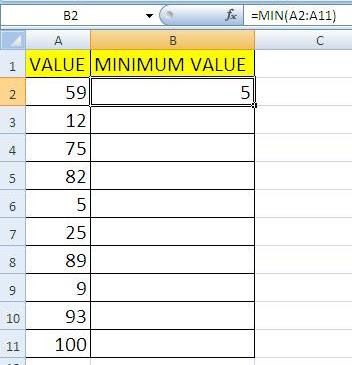### Use Excel Minimum & Maximum Formula or Function

Use Minimum, Maximum Function & Use Minimum & Maximum Function at a time.

Minimum Function:-
Excel Minimum Formula use to choose minimum value among multiples numbers. Suppose there are 10 number i.e. 59, 12, 75, 82, 05, 25, 89, 09, 93 & 100. If you want to get the minimum value among 10 numbers, use this formula in your excel cell.

Formula =MIN(cell rahge) i.e. =MIN(A2:A11) ans 5
OR USE =MIN(59,12,75,82,5,25,89,9,93,100) and 5Maximum Function:-
Excel Minimum Formula use to choose maximum value among multiples numbers. Suppose there are 10 number i.e. 59, 12, 75, 82, 05, 25, 89, 09, 93 & 100. If you want to get the maximum value among 10 numbers, use this formula in your excel cell.

Formula MAX(cell rahge) i.e. =MAX(A2:A11) ans 100
OR USE MAX(59,12,75,82,5,25,89,9,93,100) and 100Use Minimum & Maximum Function at a Time:-
When you want to insert some value within minimum & maximum value (or has lower & upper limit), you can use minimum & maximum function at a time.
Example :- I want to get excel to return a formula result only within specified minimum and maximum values. Assume between 50 to 100.

1st :- Put 75 in the A1 cell
2nd:- Then put =MIN(MAX(50,A2),100) in the call B1
3rd:- Put 200 in A1 cell, B2 cell shows maximum value 100. Put 25 in the A1 cell, B2 cell shows minimum value 50. Try more